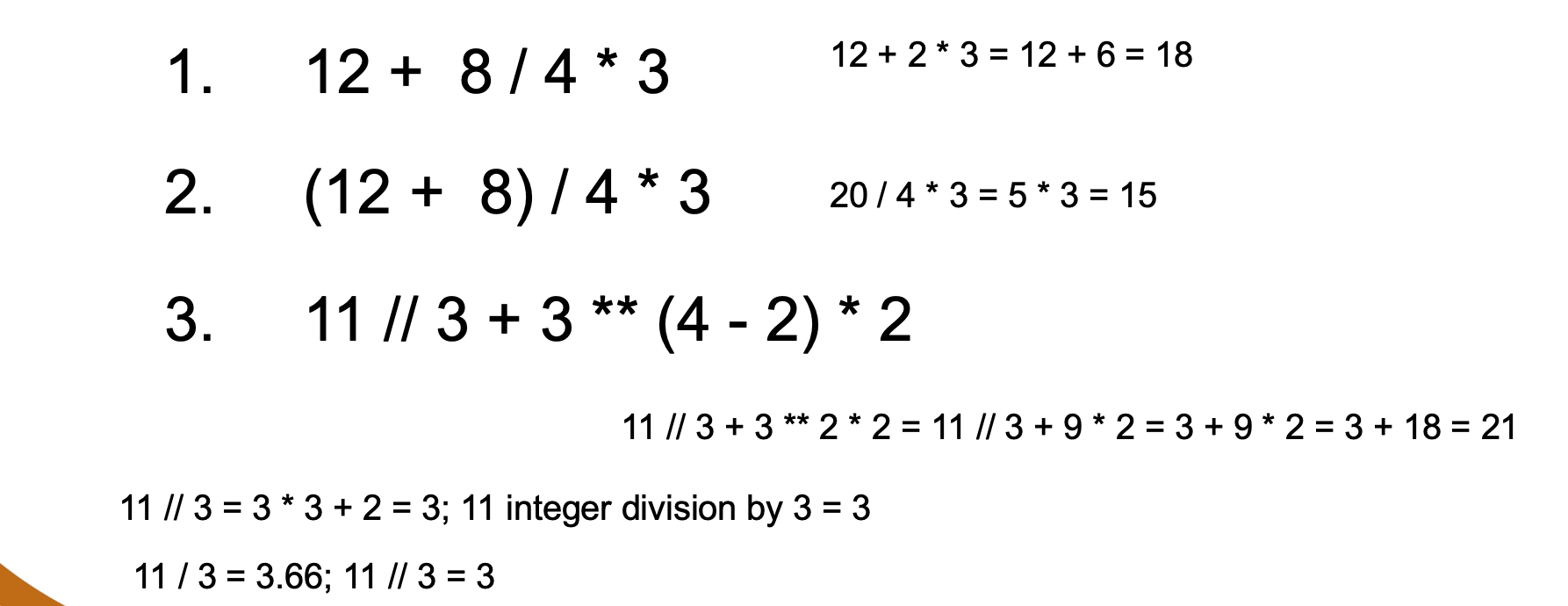# Data Types

## Data Types

• In our daily life we use numbers and text to communicate and perform certain processes.
• TThe primitive data types are numbers and text
• Objects such as images and files are also part of data types.
•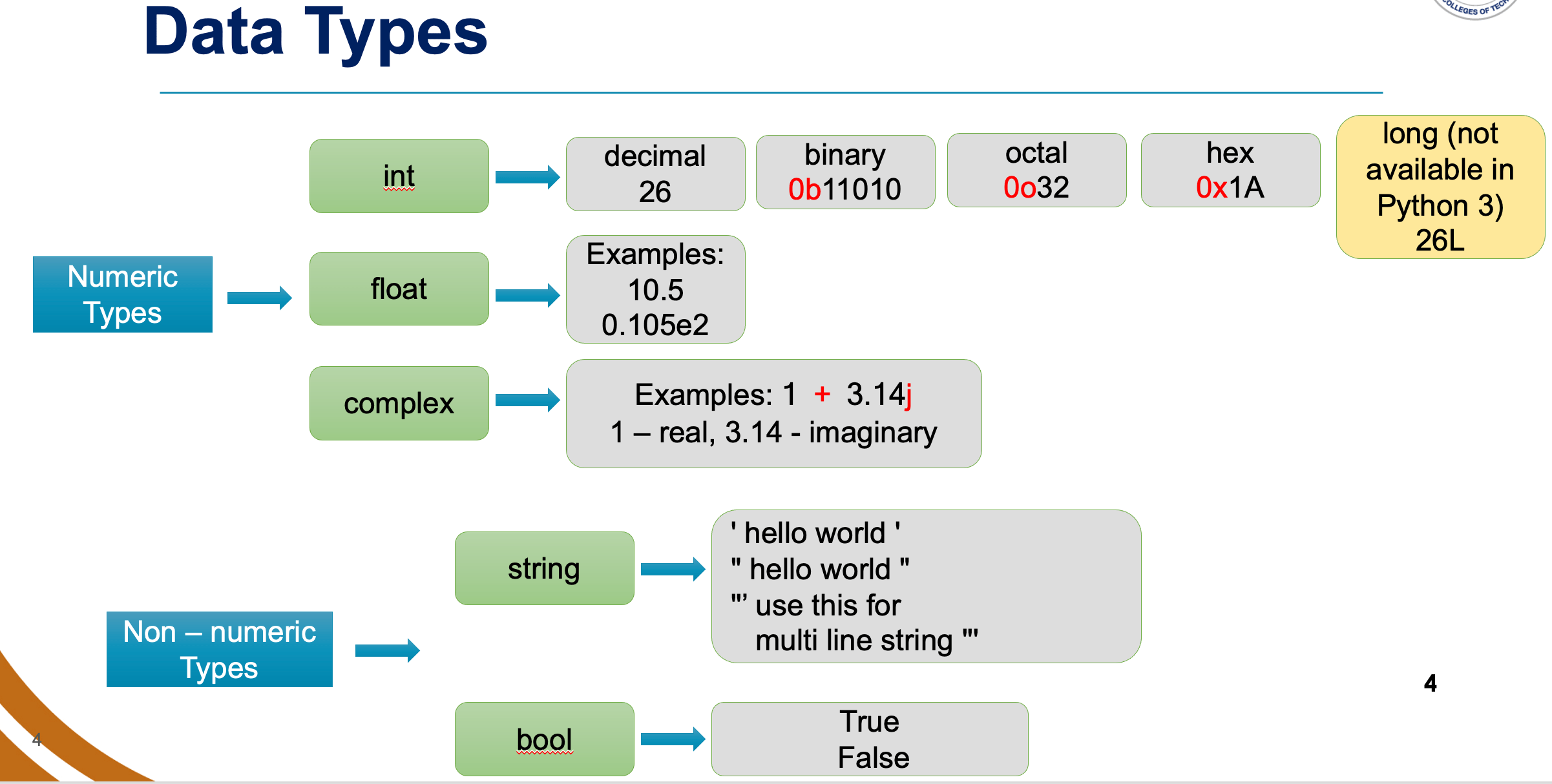### Exercise-1

Identify the data types for the following
1. Total students present in the class
2. Course code of a subject
3. Room temperature
4. NYU student ID
6. Day of the week (Sunday, Monday, etc)
7. Price of a book
8. CGPA of a student

## Variables

For example, the variable fname is used to store the first name of a person. The first name of a person is of type text or string.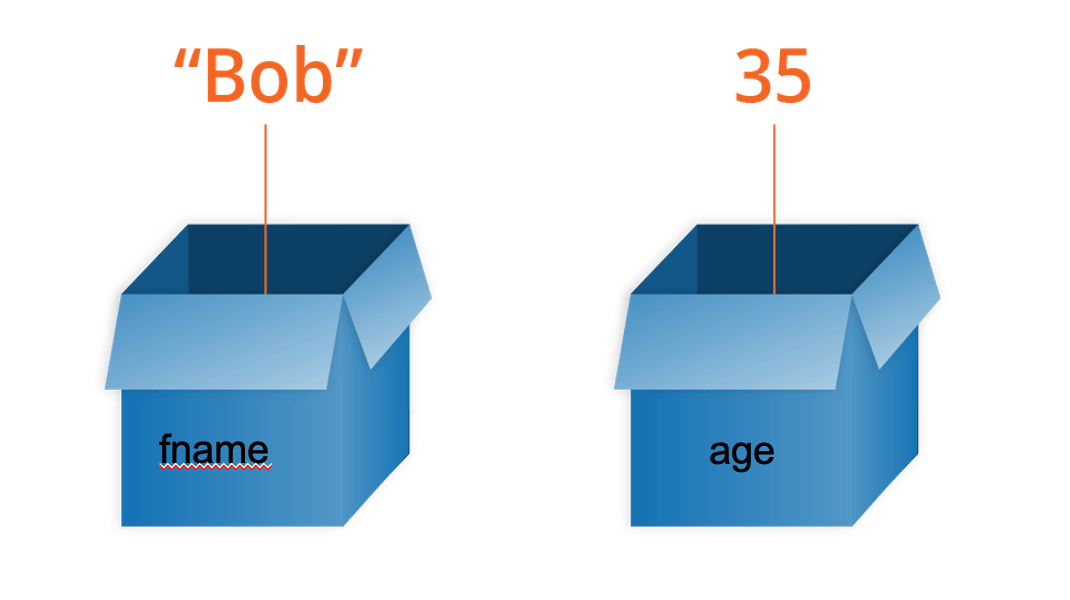## Rules for naming a Variable

• Must begin with a letter (a - z, A - Z) or (_)
• Other characters can be letters, numbers or _
• Case Sensitive. Upper case is different from lower case
• Can be any (reasonable) length
• There are some reserved words which you cannot use as a variable name because Python uses them for other things. Example: for, import, in, range

### Exercise

Identify valid variable names from the following

1. max_price
2. Total students
3. US\$
4. student_id
5. as, for, in, import (reserved words in Python)
6. discount20
7. 1st_student

### Using Variables

What if you want to change the size of the square from 100 to 50 dots?

• Solution 1: Replace all 100 by 50
• Solution 2: Use a variable instead of a value!How? X = 100
•### Using Variables, extended

• Let us go back to our square example.
• You noticed that we used 100 as the length of the square.
• Now, we will use a variable L instead of 100.
•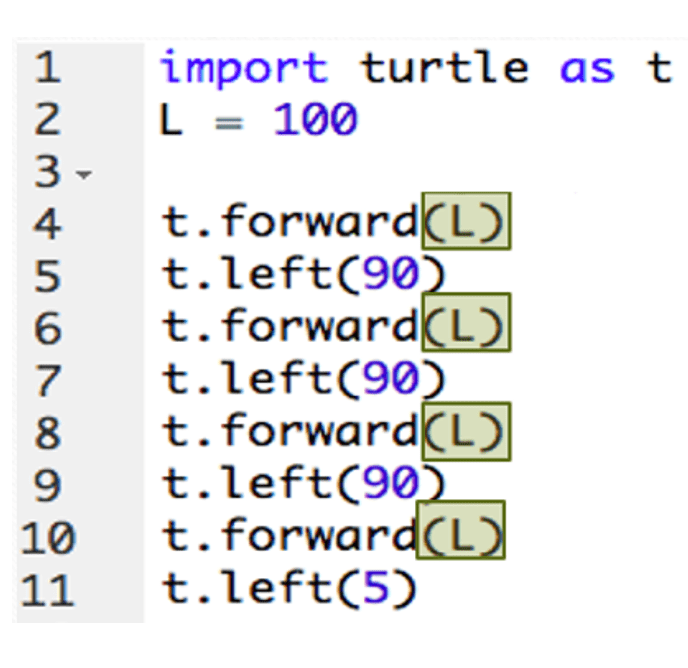## Arithmetic Operators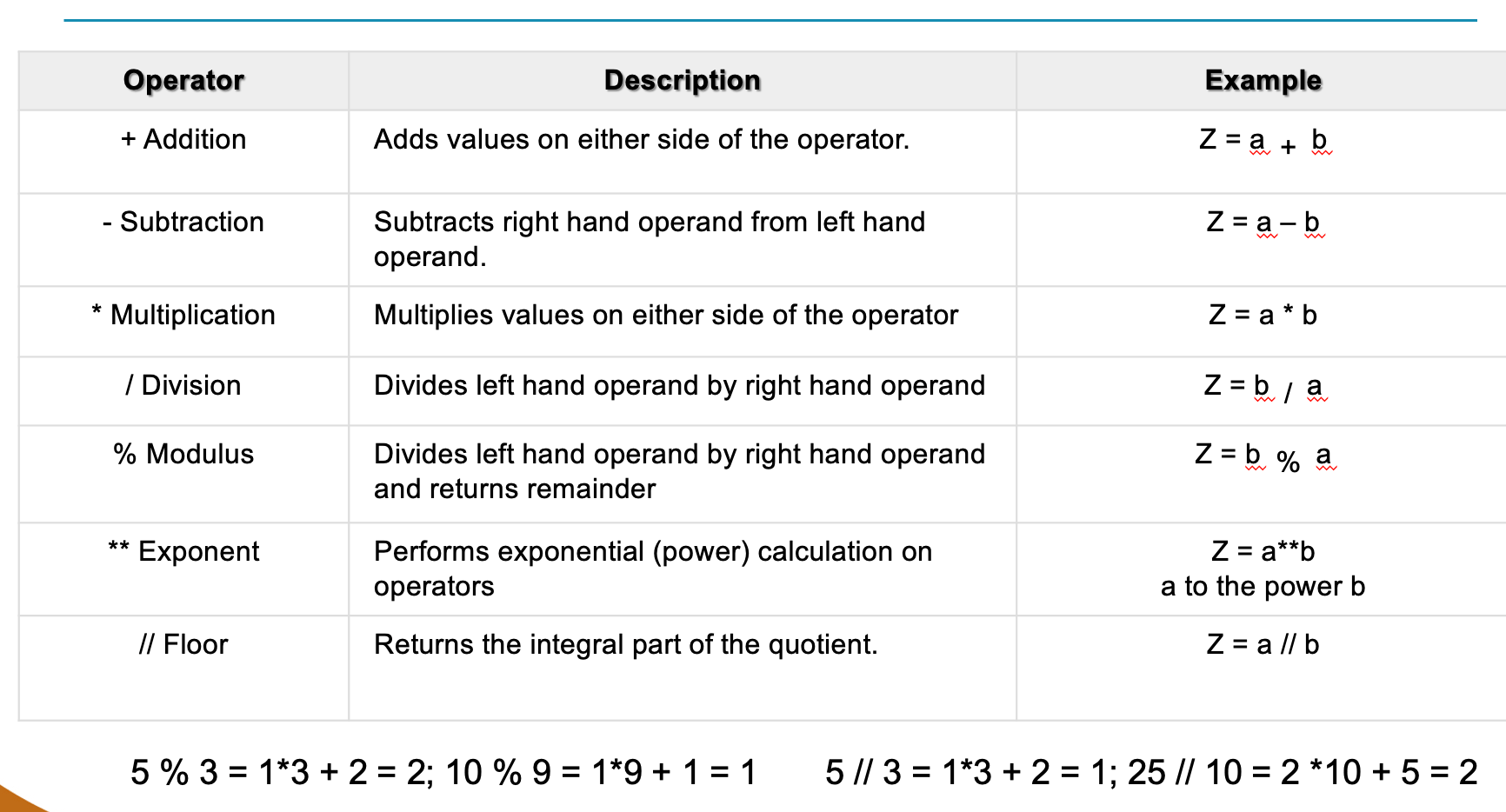### Exercise

Convert the following mathematical expression to Python expression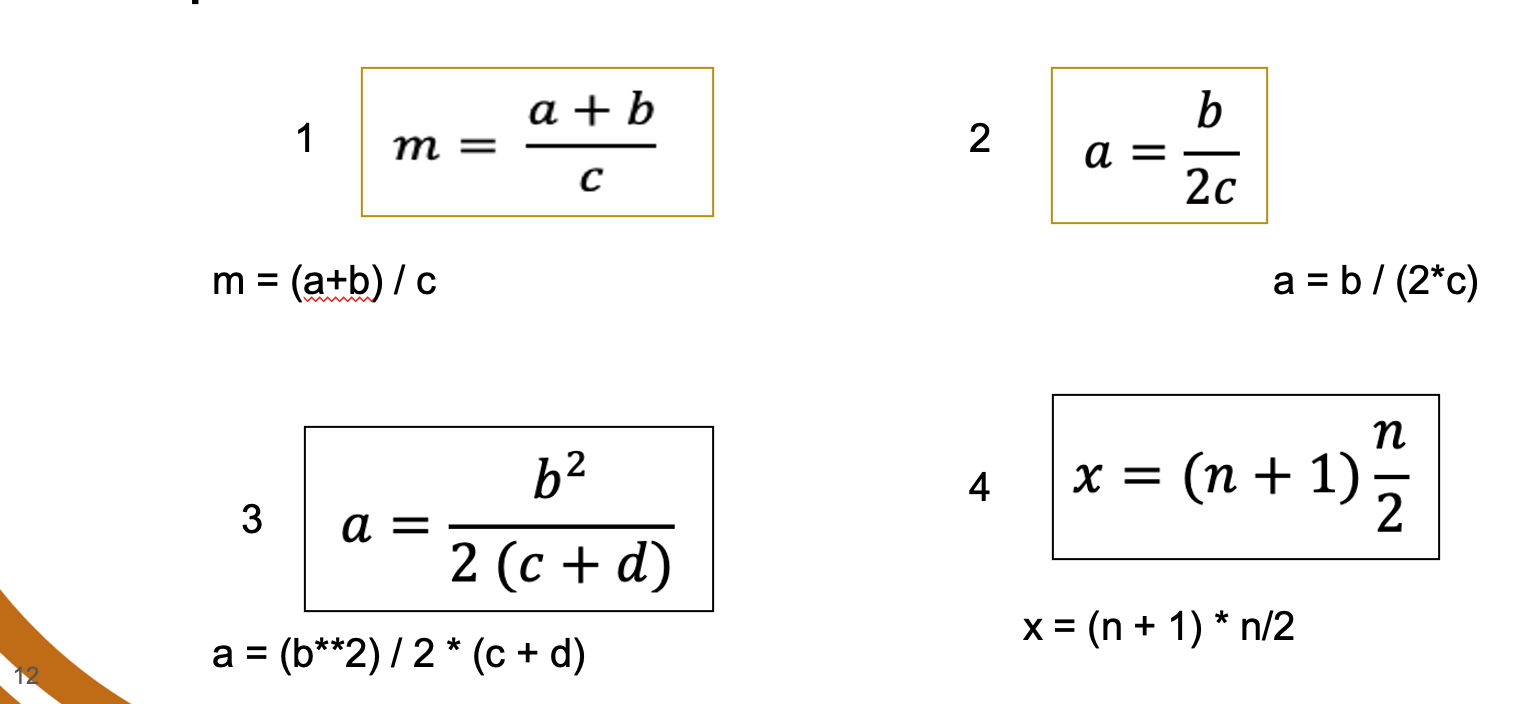## Precedence Rules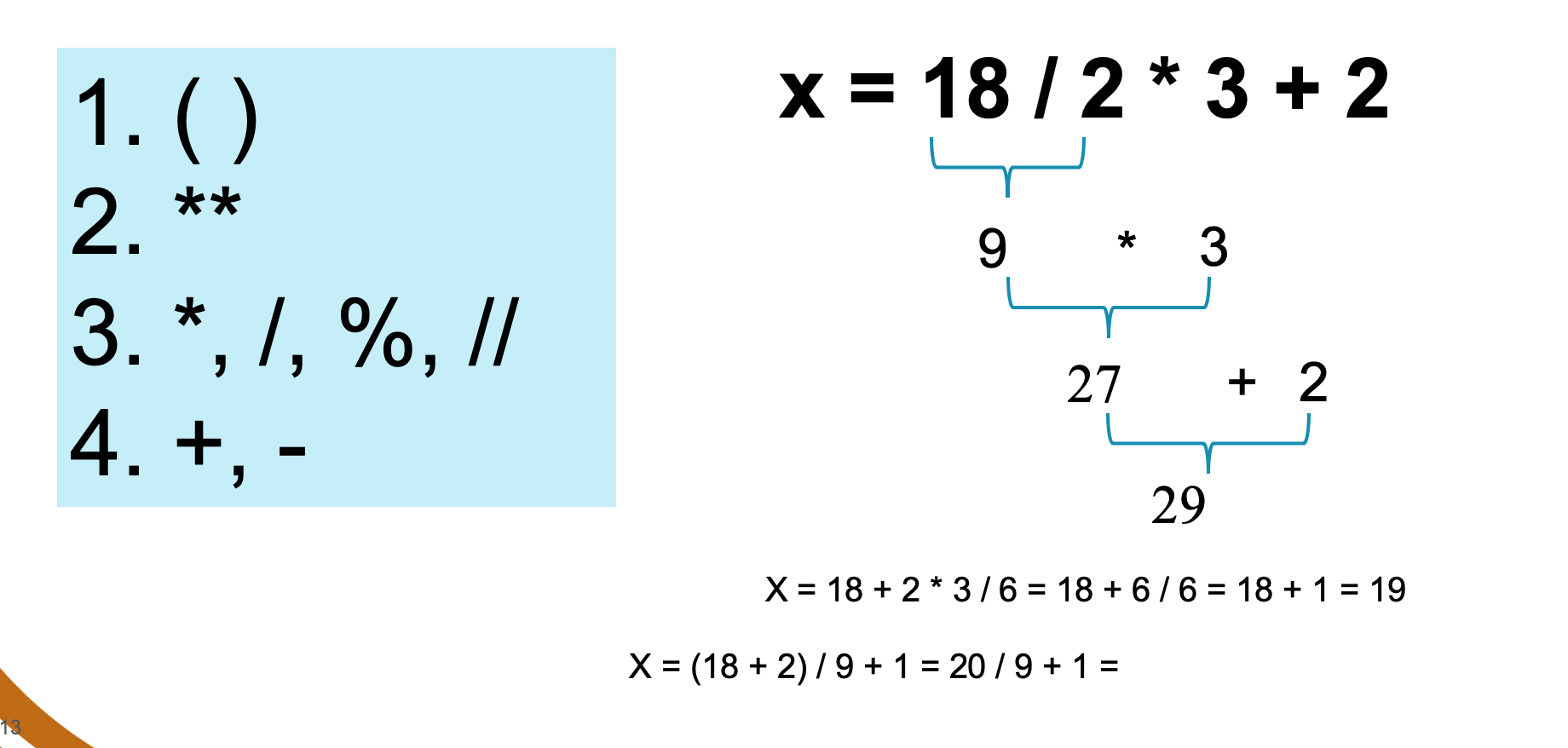### Exercise on Precedence Rules

Evaluate the following expressions using the operator precedence.